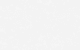# Concrete Corbel Design to ACI 318-14

## Result:

#### Reinforcement Summary:

As = 0.00 mm2, main
Ah = 0.00 mm2, horizontal

#### Checks:

• a/d = 1.0
• Nu = 0.00 kN Vu
• Vu shall not exceed of smallest of:
• φ 0.2 f'c (bw) d = 0.00 kN
• φ (3.3 + 0.08 f'c) bw d = 0.00 kN
• φ 11 (bw) d = 0.00 kN
Vu = 0.00 kN
• ε = 0.00000 < 0.004 [R1]

#### Governing values:

φ = 0.75, strength reduction factor
λ = 1.00, concrete weight factor
μ = 1.4 λ = 1.40, friction coefficient
β1 = 0.00, concrete stress block factor
Nu = 0.00 kN, design axial force
fy = 0.00 MPa (flexure & tension)
fyv = 0.00 MPa (shear friction)

#### Reinforcement computation:

• Axial Reinforcement
An = Nu/(φfy)
An = 0.00 mm2
• Shear Friction Reinforcement
Avf = Vu/(φμfyv)
Avf = 0.00 mm2
• Flexure Reinforcement [R2]
Mu = Vu(a) + Nu(h-d)
Mu = 0.00 kN-m
Af = 0.00 mm2
• Required Main Reinforcement
As shall be the largest of:
• Af + An = 0.00 mm 2
• (2/3) Avf + An = 0.00 mm 2
• 0.04(f'c/fy)bw d = 0.00 mm 2
As = 0.00 mm2
• Required Horizontal Reinforcement
Ah = 0.5 (As - An)
Ah = 0.00 mm2

## Background:

### Description

A corbel is a short cantilever projecting out from a column, a wall, or the side of a beam. A corbel is designed to support another member, usually a beam or girder, but may also be used to support a slab. ACI 318 restricts the term “corbel” to a member having span-to-depth ratio which is less than or equal to 1.0. Corbels are widely used in precast concrete construction.

This technical background discusses the requirements for the design of a reinforced concrete corbel in both metric version ACI 318M-14 and the US customary unit version ACI 318-14 of the code. Formulas and terms are primarily expressed in metric units with the equivalent US unit enclosed in square brackets [ ]. The online calculator allows the engineer to select the desired unit.

### Geometric Requirements

Chapter 16.5 of the ACI code specifies the provisions for the design of brackets and corbels. Corbels are commonly trapezoidal-shaped but may also be rectangular. For trapezoidal-shaped corbels, the outer depth at the edge of the corbel should have minimum dimension of 0.5 x (effective depth) as depicted in the cover image. The span-to-depth ratio a/d should not exceed 1.0. This is the limit of the tests that were conducted in the formulation of equations.

For normal-weight concrete, according to Section 16.5.2.4, the nominal vertical load Vu/φ should not exceed the smallest of:

• 0.2f’cbwd                    [ 0.2f’cbwd ]
• (3.3 + 0.08f’c)bwd     [ (480 + 0.08f’c)bwd ]
• 11bw d                        [ 1600bwd ]

For light-weight concrete, according to Section 16.5.2.5, Vu/φ should not exceed the smallest of:

• (0.2 – 0.07a/d)f’cbwd     [ (0.2 – 0.07a/d)f’cbwd ]
• (5.5 – 1.9a/d)bwd           [ (800 – 280a/d)bwd ]

For convenience in the online calculation, the terms above are multiplied by the strength reduction factor φ and compared directly with Vu. The strength reduction factor φ is taken as 0.75 as per Table 21.2.1(f) applicable for brackets and corbels. The reduction factor of 0.75 is used for flexure, axial, and shear-friction strength evaluation.

• Maximum horizontal force: Nu ≤ Vu              — Section 16.5.1.1
• Minimum horizontal force: Nu ≥ 0.2Vu        — Section 16.5.3.5

The minimum horizontal force may be ignored if there are other means to prevent the tensile forces from being transferred into the corbel, such as providing horizontally sliding bearing supports. The online calculator gives the option to ignore the minimum Nu=0.2Vu, e.g. you may use 0 as input value.

### Design Property of Materials

The design strength of reinforcement is capped to maximum value of fy = 550 MPa [80 ksi] for flexure and fyv = 420 MPa [60 ksi] for shear-friction as per Table 20.2.2.4a.